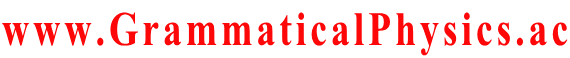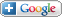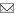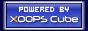Welcome Guest
www.GrammaticalPhysics.ac

Access Detector
3 user(s) are online (2 user(s) are browsing Forum)

Members: 0
Guests: 3

more...

# Discretized Classical Model of the New Equation

SourceCodeOf_HumanGenome > Discretized Classical Model of the New Equation @ 2014/5/13 17:31
H(p;x) = [1／(2M)]Σk[pk - QAk(x)]2 + Qφ(x).
M = mεα2, Q = m.
Ak(x) = α(xk+1 - xk),
φ(x) = -[1／(2ε)]Σk(xk+1 - xk)2.

dxj/dt = ∂H/∂pj = (pj - QAj)／M,
dpj/dt = -∂H/∂xj = (Q/M)Σk(pk - QAk)∂Ak/∂xj - Q∂φ/∂xj.

pj = Mdxj/dt + QAj,
M(d/dt)2xj + QdAj/dt
= (Q/M)Σk(pk - QAk)∂Ak/∂xj - Q∂φ/∂xj
= (Q/M)Σk(Mdxk/dt)∂Ak/∂xj - Q∂φ/∂xj,
dAj/dt = Σk(∂Aj/∂xk)(dxk/dt),
∴ M(d/dt)2xj = (Q/M)Σ(Mdxk/dt)∂Ak/∂xj - QΣ(∂Aj/∂xk)(dxk/dt) - Q∂φ/∂xj,
∴ M(d/dt)2xj = QΣk(dxk/dt)[∂Ak/∂xj -∂Aj/∂xk] - Q∂φ/∂xj.

M(d/dt)2xj = Qα(dxj-1/dt - dxj+1/dt) + Q(2/ε){xj - (1/2)[xj-1 + xj+1]}.
This equation is different from the following equation which represents an automatic collision avoidance system.
M(d/dt)2xj = Qα[(dxj-1/dt - dxj/dt) + (dxj+1/dt - dxj/dt)] - Q(2/ε){xj - (1/2)[xj-1 + xj+1]}.

Multiplying both sides of the equation by dxj/dt and summing over j, we get the following equation representing the energy conservation law.
(d/dt)Σa≦j≦b(M/2)(dxj/dt)2 = Qα[(dxa-1/dt)(dxa/dt) - (dxb/dt)(dxb+1/dt)] + Q(1/ε)(d/dt)Σa≦j≦b-1[(xj)2 - xjxj+1] - (Q/ε)(xa-1dxa/dt + xb+1dxb/dt) + (Q/ε)(d/dt)(xb)2.
∴ (d/dt)[Σa≦j≦b(M/2)(dxj/dt)2 - Σa≦j≦b-1(Q/ε)xj(xj - xj+1)]
= Q(dxa/dt)(αdxa-1/dt - xa-1/ε) - Q(dxb/dt)(αdxb+1/dt + xb+1/ε) + (Q/ε)(d/dt)(xb)2.
∴ (d/dt)[Σa≦j≦b(M/2)(dxj/dt)2 - Σa≦j≦b-1(Q/ε)xj(xj - xj+1) - (Q/ε)(xb)2]
= Q(dxa/dt)(αdxa-1/dt - xa-1/ε) - Q(dxb/dt)(αdxb+1/dt - xb+1/ε) - 2(Q/ε)(dxb/dt)xb+1.

I want to let the equation reduce to the form meaning that energy of a≦j≦b varies by energy flow at j=a and j=b.
However the term - 2(Q/ε)(dxb/dt)xb+1 prevents it.
It is of course because of the interaction between j=a-1 and j=a and the interaction between j=b and j=b+1.
An equation of such a form may be made in the continuous case.

To know the character of this problem, I omit the scalar potential term and try solving the following equation.
M(d/dt)2xj = Qα(dxj-1/dt - dxj+1/dt).

M(d/dt)2xj = QαΣkAj,kdxk/dt,
Aj,k = δj-1,k - δj+1,k

dx/dt = a[exp(QαtA/M)]b
where a is a real number, and b is an infinite column vector.

(A2)j,k
= ΣsAj,sAs,k
= Σsj-1,s - δj+1,s)(δs-1,k - δs+1,k)
= δj-2,k - 2δj,k + δj+2,k

(A3)j,k
= Σsj-2,s - 2δj,s + δj+2,s)(δs-1,k - δs+1,k)
= δj-3,k - 3δj-1,k + 3δj+1,k - δj+3,k

(A4)j,k
= Σsj-3,s - 3δj-1,s + 3δj+1,s - δj+3,s)(δs-1,k - δs+1,k)
= δj-4,k - 4δj-2,k + 6δj,k - 4δj+2,k + δj+4,k

(A5)j,k
= Σsj-4,s - 4δj-2,s + 6δj,s - 4δj+2,s + δj+4,s)(δs-1,k - δs+1,k)
= δj-5,k - 5δj-3,k + 10δj-1,k - 10δj+1,k + 5δj+3,k - δj+5,k

(An)j,k
= Σ0≦s≦n/2(-1)sc1(s,n)δj-n+2s,k + Σ0≦s＜n/2(-1)n+sc2(s,n)δj+n-2s,k,
cr(s,n+1) = cr(s,n) + cr(s-1,n),
cr(0,n) = 1,

If a = 1, bj = δj,0 then:
dxj/dt = Σn=0(1/n!)(Qαt/M)n0≦s≦n/2(-1)sc1(s,n)δj-n+2s,0 - Σ0≦s＜n/2(-1)sc2(s,n)δj+n-2s,0].

From the point of view of energy conservation, velocity does not increase or decrease as a whole.
This is expected to be represented well by
dx/dt = a exp(it×somematrix) b,
but the above solution does not include the imaginary unit.
Perhaps the matrix A has a property like a pure imaginary number.

---
Last edited at 2014/05/15/16:53JST
Site Search
 Grammatical Physics SearchGadgets powered by Google
Site Info
Webmasterswooder